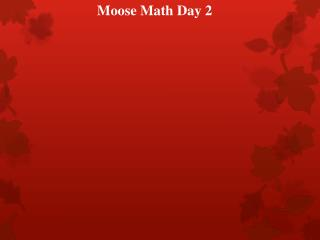DownloadDownload PresentationMoose Math Day 2

# Moose Math Day 2

Télécharger la présentation## Moose Math Day 2

- - - - - - - - - - - - - - - - - - - - - - - - - - - E N D - - - - - - - - - - - - - - - - - - - - - - - - - - -
##### Presentation Transcript

1. Moose Math Day 2

2. Ten Minute Math Write the following numbers in EXPANDED FORM! 648 1.508 908,467

3. Ten Minute Math Answers! 648 = 600 + 40 + 8 1.508 = 1,000 + 500 + 8 908,467 = 900,000 + 8,000 + 400 + 60 + 7

4. Subtraction Starter Problems Today you are going to spend the first part of class working in pairs to solve three starter problems in each set and then choose one of those starts to solve a final subtraction problem. You should help each other solve the starter problems mentally if possible and then, after you have chosen a start, decide together how you will finish the solution to the problem. Record your work on NOTEBOOK paper.

5. Do I Add or Subtract? Let’s look at the first STARTED problem for problem 1: 97-40 Did anyone use this starter to solve for 97-39? If so, how did you finish the problem? What would you do after 97-40? If needed, here are some examples other students used to finish this problem:

6. Do I Add or Subtract?

7. Do I Add or Subtract?

8. Examining the U.S. Algorithm Independent Work: MM p. 47-48